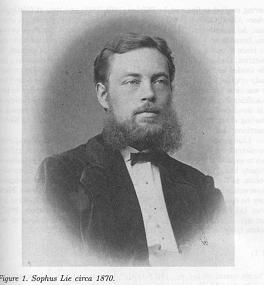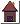# PhD interests

### Semisimple Lie algebras

Lie algebras were introduced toward the end of nineteenth century in order to study some problems arising from geometry. In the interest of classifying these objects, the subcategory of semisimple Lie algebras has been studied. This is the class of algebra in which I am interested in. The easiest example of such algebra is almost given by 𝖌=𝖌ln, the set of matrices of size n equiped with the Lie bracket [A,B]=A.B-B.A. Such an algebra is in fact called reductive since only [𝖌,𝖌] is semisimple.

### Symmetric Lie algebras

There exists a well known classification of complex semisimple Lie algebras (An, Bn, Cn, Dn, E6, E7, E8, F4 et G2). When the groundfield is real, the classification is heavily based upon the complex one. Concretely, let 𝖌 be a real semisimple Lie algebra and let θ be its Cartan involution. Then, decomposing 𝖌 into θ-eigenspaces respectively associated to +1 and -1, one gets 𝖌=𝖐+𝖕. This decomposition is linked with the decomposition of any real Lie group into compact and non-compact part. For instance the Polar decomposition is such a decomposition when 𝖌=𝖌ln.

When one complexifies these notions, one gets a pair (𝖌) associated to a decomposition 𝖌=𝖐+𝖕. Then 𝖐 is a (reductive) Lie subalgebra of 𝖌 and 𝖕 is an ad 𝖐-module where ad(x)=[x,.] is the Lie algebra adjoint action. A pair (𝖌,θ) is called a symmetric Lie algebra when 𝖌 is a Lie algebra and θ is an involution of 𝖌.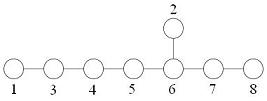The above described method yields to a one to one correspondance between real semisimple Lie subalgebras and complex symmetric Lie subalgebras. This correspondance is made deeper by the so-called Kostant Sekiguchi correspondance. The latter establishes a bijection between nilpotent orbits of 𝖌 and nilpotent orbits of 𝖕.

A particular case of symmetric Lie algebra is when 𝖌 is isomorphic to the direct sum of two copies 𝖌0 and 𝖌1 of a Lie algebra 𝖌', and when θ(𝖌i)=𝖌1-i, i=0,1. Then, the Lie algebra 𝖐={x+θ(x)| x∈ 𝖌1} is isomorphic to 𝖌' while the ad 𝖐-module 𝖕={x-θ(x)| x∈ 𝖌1} is isomorphic to the ad 𝖌'-module 𝖌'. That's why symmetric Lie are often considered as a generalization of Lie algebras. Furthermore, lots of notions arising from Lie theory (e.g. Cartan subalgebras, root systems, Dynkin diagrams, 𝖘l2-triples,...) have a symmetric generalization (e.g. Cartan subspaces, restricted root systems, Satake diagrams, normal 𝖘l2-triples,...).

### Geometry in Lie algebras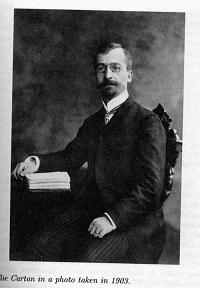There is an another level structure on (semisimple complex) Lie algebras. Denoting by G the algebraic adjoint group of 𝖌, we can consider 𝖌 as a G-variety under the adjoint action Ad. We can then study some properties in the framework of algebraic geometry.

For instance, the notion of semisimple or nilpotent element of 𝖌 can be defined geometrically. Semisimple elements are elements whose (G-)orbit is closed, while nilpotents elements are elements whose G-orbit contains 0 in its closure. Again, this structure has a symmetric analogue. Indeed, one can define the connected subgroup K of G having 𝖐 as Lie algebra. Then, 𝖕 is an Ad K-variety.

One can then study various G-varieties arising from this setting. From a global perspective, I try to generalize or understand some properties of analogue varieties in symmetric Lie algebras

### Example 1: The commuting variety

As a first exemple, we can talk about the commuting variety 𝕮(𝖌):={(x,y)∈𝖌×𝖌| [x,y]=0}. This variety is irreducible (Richardson 1979). Similarily, it has been recently shown (Premet 2003) that the nilpotent commuting variety 𝕮nil(𝖌)=𝕮(𝖌)∩𝓝×𝓝 is equidimensional and that its irreducible components are indexed by so-called distinguished nilpotent orbits.

From a symmetric point of view, one should speak about the study of the symmetric commuting variety 𝕮(𝖕)=𝕮 (𝖌)∩(𝖕×𝖕). In the general case, It is not irreducible nor equidimensionnal. Personnally, I have been interested in the study of the symmetric nilpotent commuting variety 𝕮nil(𝖕)=𝕮nil(𝖌)∩(𝖕×𝖕). In 15 out of 20 cases, I could prove the following: 𝕮nil(𝖕) is equidimensional.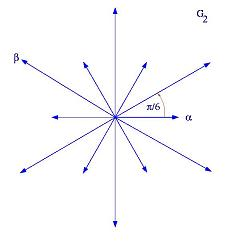### Example 2: Sheets

Sheets (or G-sheets) are some other examples of varieties already studied. These are irreducible components of subsets of the form 𝖌(m):={x∈𝖌| dim G.x=m}; m∈ ℕ. The study of these varieties is linked with various geometric problems arising in Lie theory. For instance, the proof by Richardson of the irreducibility of the commuting variety is based upon some results about sheets.

A part of the study of sheets has been made through an object called Slodowy Slice. When (e,h,f) is an 𝖘l2-triple such that e belong to a sheet S, a Slodowy slice of S is e+X:=S∩(e+𝖌f). In particular, one can show that S=G.(e+X) and that a geometric quotient of S can be expressed as a quotient of X by a finite group.

The K-sheets are a good symmetric analogue to sheets. They are defined as irreducible components of subsets of the form 𝖕(m):={x∈ 𝖕| dim K.x=m}, m∈ℕ. In my study of these objects, I could show that the parametrization through the Slodowy slice still had some good properties when 𝖌=𝖌ln. In particular, it has been possible to prove that K-sheets are the regular elements of the closure of sets of the form K.(e+X∩𝖕) when (e,h,f) is a normal 𝖘l2-triple.

 - Cool non? - Michaël Bulois         Last update: 17 December 2011 - nice website, isn't it?-